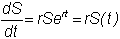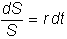﻿ 6.6 Fisher-Weil Durationoffice (412) 9679367
fax (412) 967-5958
toll-free 1 (800) 214-3480

Appendix D  Chapter 6 Technical Topic:  Stock Price Dynamics

D.1  Stock Price Dynamics:  Certainty Model

 A

ssume that the stock price S grows at the continuously compounded rate r  in a market with no uncertainty.  The equation describing this growth has the following form:If you differentiate this with respect to t, you getThus, the certainty model assumes that the rate of change of the stock price through time is a constant proportion of the current stock price.

This expression describes the dynamic stock price process in a world with certainty.  Multiplying both sides by dt and rearranging,  we can rewrite the equation as:In this form, the instantaneous rate of return on the stock is r.  Here, r is also called the drift rate of the stock price process.   In the technical topic Ito Processes, the dynamic stock price process presented in this current topic is extended to a world with uncertainty.  In this extension, a constant volatility term is added so that the price process is assumed to follow a specific type of diffusion process.